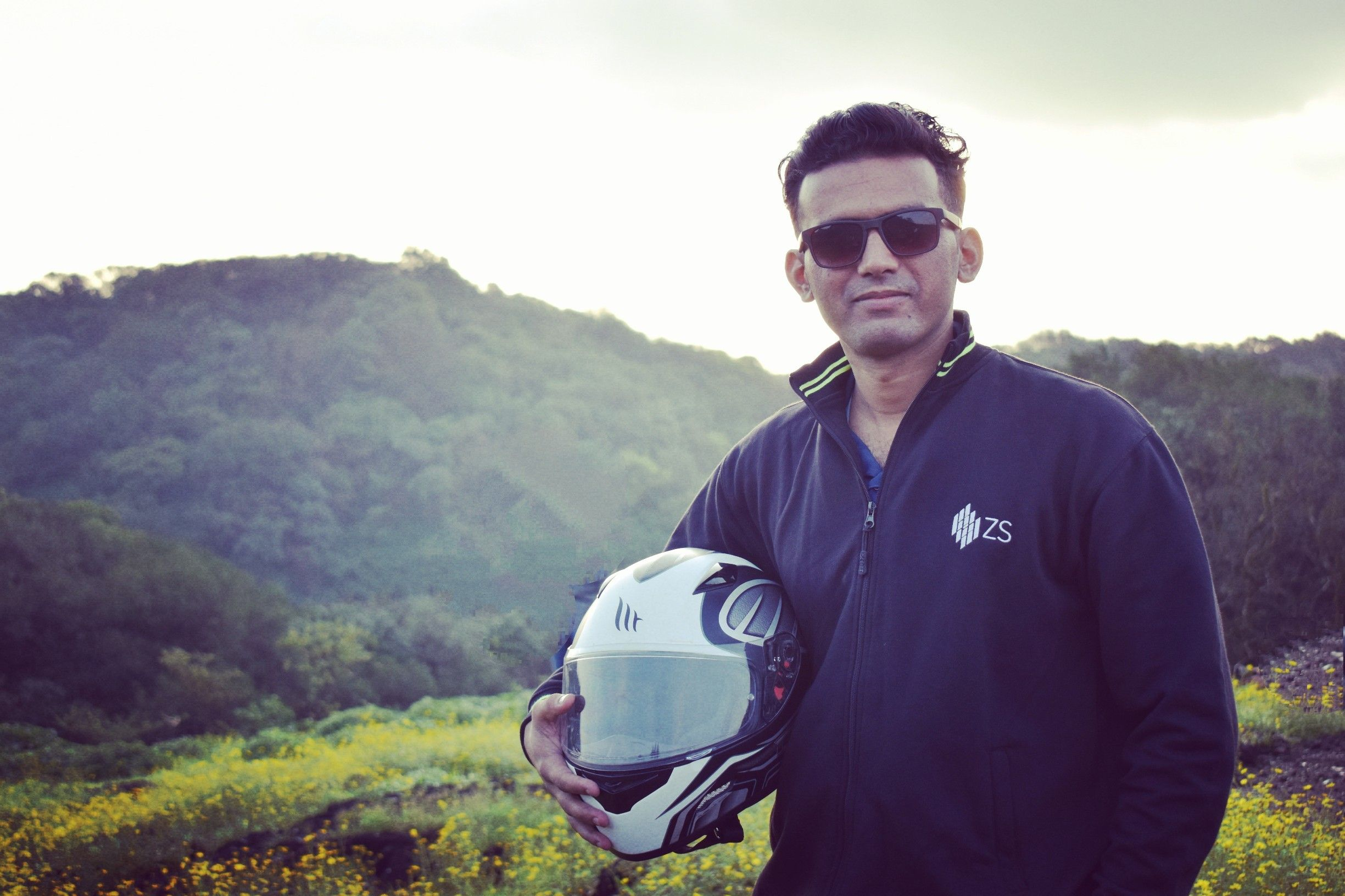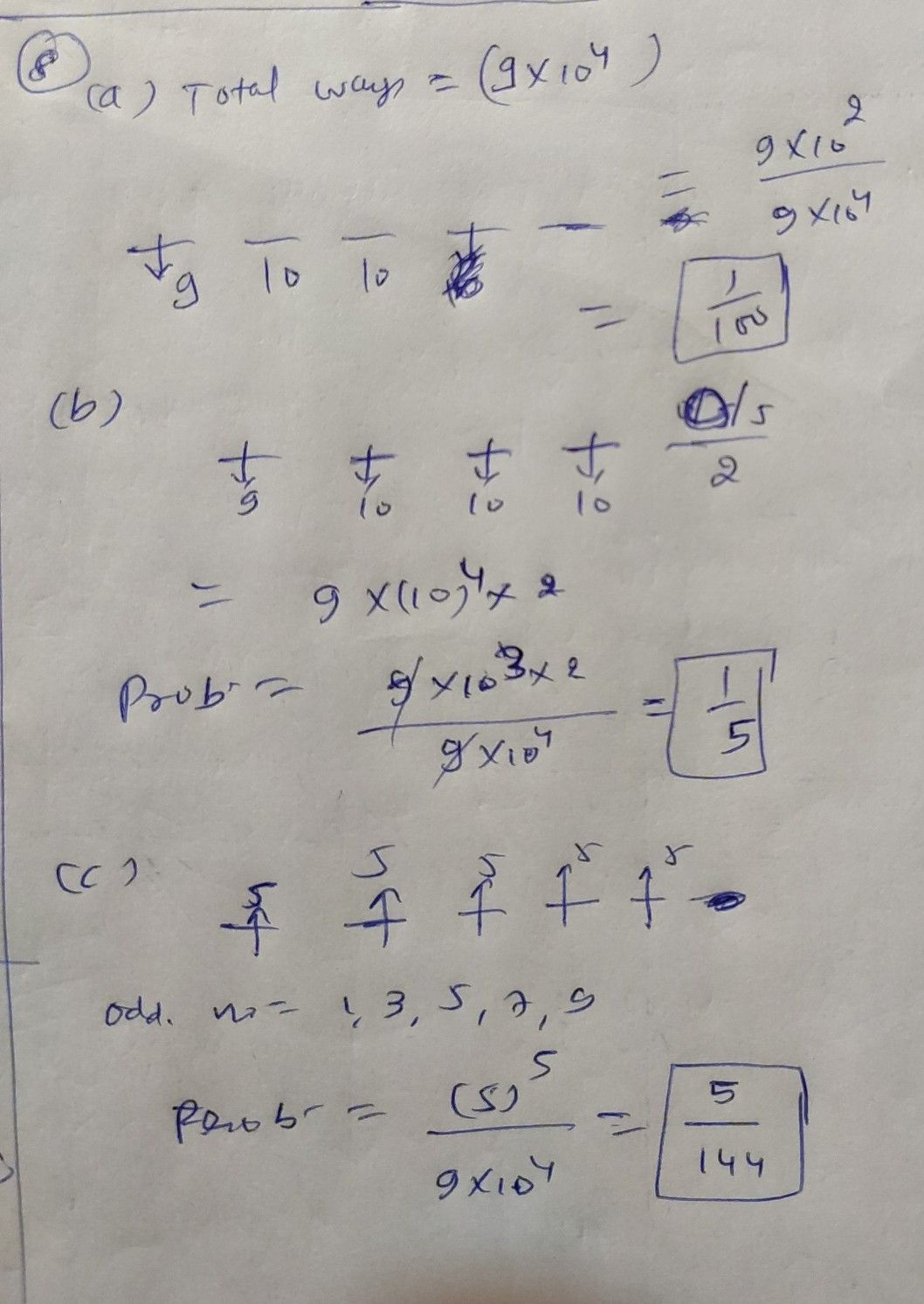Symbol
Problem$sOO$ on. $1$ even. $8$ $A$ five-digit number is chosen at random. What is the probability of the following events $:A$ $=1$ {the number reads alike from left to right and from right to left (as number $13531\right)\right)$ $B=\left(th$ $5$ number is multiple of five}, $C=11h$ {the number consists of odd digits}.